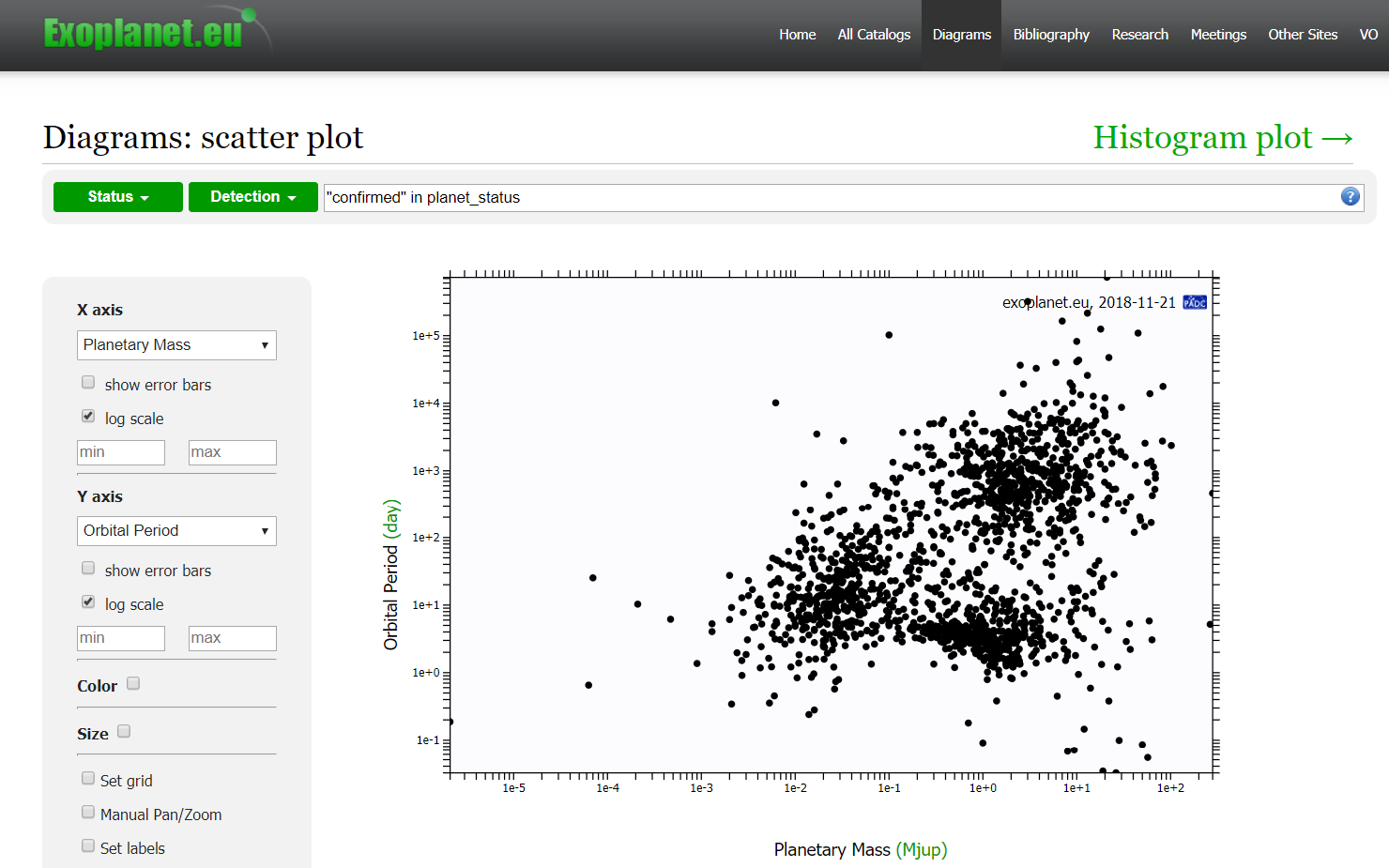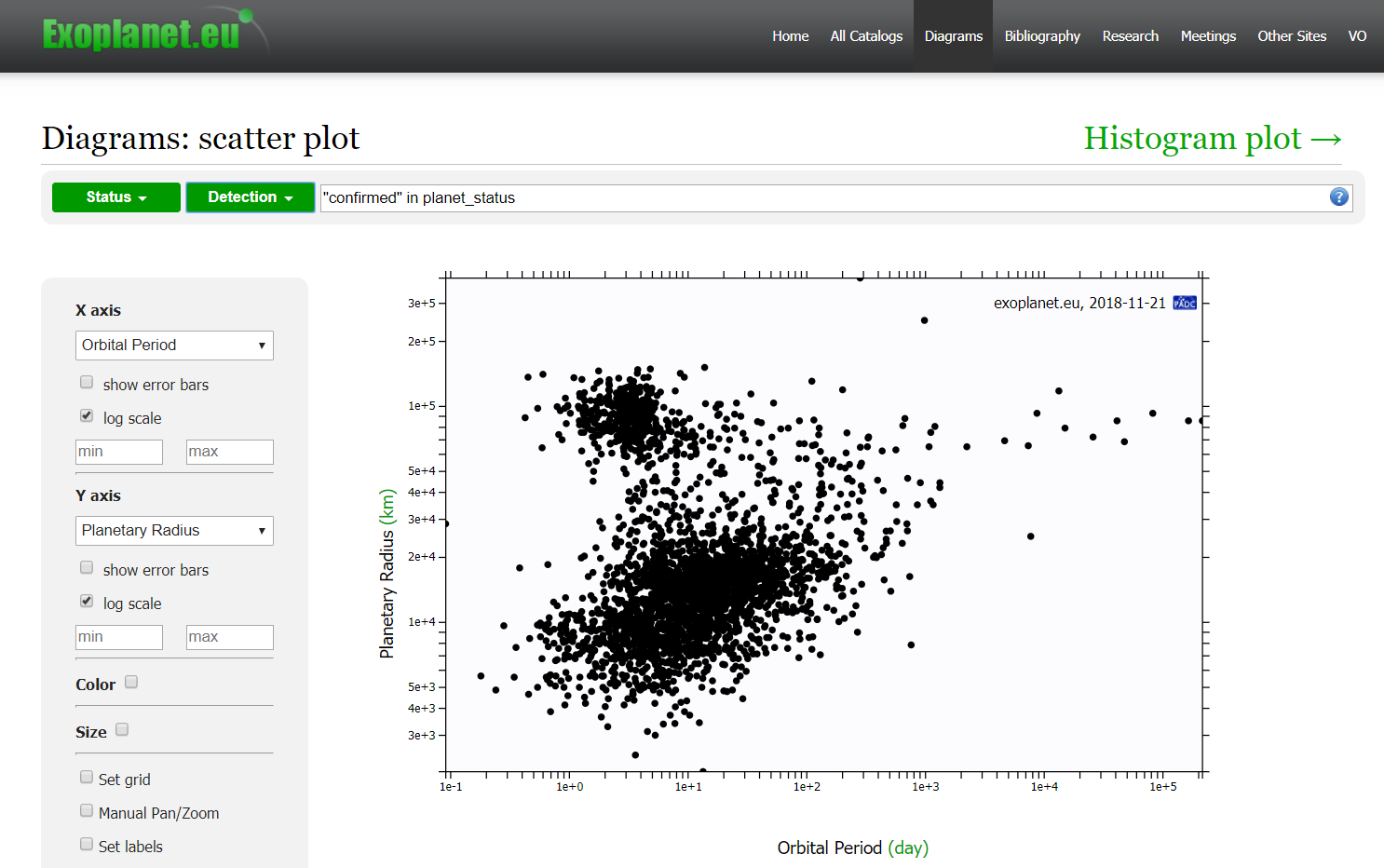# The Exoplanet Activity

This activity makes use of the The Extrasolar Planets Encyclopaedia and the plots that you can create on that website to help you to understand more about the properties of exoplanets.

To start the activity, you'll need to go to this page.Figure 1: A scatter plot of Planetary Mass against Orbital Period Credit: exoplanet.eu

On this page (see Figure 1), you'll see lots of dropdown menus but the two you may want to start with are under the labels "X axis" and "Y axis". The defaults here are "Planetary Mass" and "Orbital Period" and you'll notice that the "log scale" boxes are ticked. This means that the axes are shown in logarithmic (rather than linear) form allowing us to show a broad range of values more easily on a single plot. For examples, the masses of the planets shown on this default plot range from ~ 0.02 up to ~ 90,000 Earth masses, values which are almost impossible to plot meaningfully with a linear scale.

Your task is to try to identify trends or concentrations within the plots. You have 36 parameters to choose from giving you a total of 1260 (36 * 35) plots which you can create. You may want to predict what a particular set of values might look like before creating the graph.

Trends in your data may take the form of a straight (or nearly straight; astronomical data do not always behave perfectly) line. An example of this might be plotting the magnitudes of the host stars in two different filters. For example, try plotting J against H magnitudes (these are infrared observations) using linear scales. This shows a very clear trend from bottom left to top right. To confuse the matter a little here, the magnitude scale is inverted so lower numbers represent brighter stars than high numbers. The brightest stars are therefore in the lower left of this plot. There are a handful of values where the J and H magnitudes are a little different but generally, the values are the same to one or two significant figures.

Sometimes, the data may show a trend in the opposite direction so that as one value increases, the other decreases. These properties would be considered to be inversely proportional. You could try to find some examples of this within this activity.Figure 1: A scatter plot of Orbital Radius against Planetary Radius Credit: exoplanet.eu

Finally, you may see areas of your plot where the data seem to be more concentrated (see Figure 2). An example is the plot of Orbital Period against Planetary Radius using log scales on both axes. Here, the data are seen in two distinct areas within the plot. What do you think these two areas might represent? (hint: think about the distribution of planets in our own Solar System)

Within the activity, there may be occasions where you discover what's known as a selection effect. This is a form of bias where a particular choice of data or parameters produces a false representation of what's happening. For example, if you wanted to estimate how many people in Great Britain like football, you might not get an unbiased representation by conducting your survey near a football ground at 2PM on a Saturday !

We provide some examples of selection effects. You can also view or post on the discussion forum.